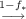#### Vol. 106, No. 1, 1983

 Recent Issues Vol. 323: 1  2 Vol. 322: 1  2 Vol. 321: 1  2 Vol. 320: 1  2 Vol. 319: 1  2 Vol. 318: 1  2 Vol. 317: 1  2 Vol. 316: 1  2Online Archive Volume: Issue:The Journal Subscriptions Editorial Board Officers Contacts Submission Guidelines Submission Form Policies for Authors ISSN: 1945-5844 (e-only) ISSN: 0030-8730 (print) Special Issues Author Index To Appear Other MSP Journals
On the computations of the Nielsen number

### Boju Jiang

Vol. 106 (1983), No. 1, 105–113
##### Abstract

The aim of this note is to show the following

Main Theorem. Let X be a compact connected ANR, f : X X be a map. Suppose there is an integer n such that fπn(π1(X)) J(fn). Then any two fixed point classes of f have the same index. Hence

 L(f) = 0 implies N(f) = 0, while L(f)≠0 implies N(f) = ♯Coker(H1(X)H1(X)).
Here L(f) and N(f) are the Lefschetz number and Nielsen number of f respectively, and J(F) π1(X) stands for the Jiang subgroup of f.

Primary: 55M20
##### Milestones